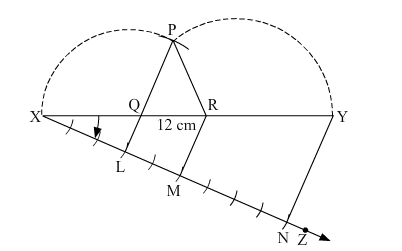# Construct a ∆PQR whose perimeter is 12 cm and the lengths of whose sides are in the ratio 3 : 2 : 4.

Question:

Construct a PQR whose perimeter is 12 cm and the lengths of whose sides are in the ratio 3 : 2 : 4.

Solution:1. Draw a line segment XY = 12 cm.
2. In the downward direction, construct an acute angle with XY at X.
3. From X, set off (3 + 2 + 4) = 9 arcs of equal distances along XZ.
4. Mark points L, M and N such that XL = 3 units, LM = 2 units and MN = 4 units.
5. Join NY.
6. Through L and M, draw LQ "> NY and MR "> NY cutting XY at Q and R, respectively.
7. With Q as the centre and radius QX, draw an arc.
8. With R as the centre and radius RY, draw an arc, cutting the previously drawn arc at P.
9. Join PQ and PR.

Thus, $\triangle P Q R$ is the required triangle.